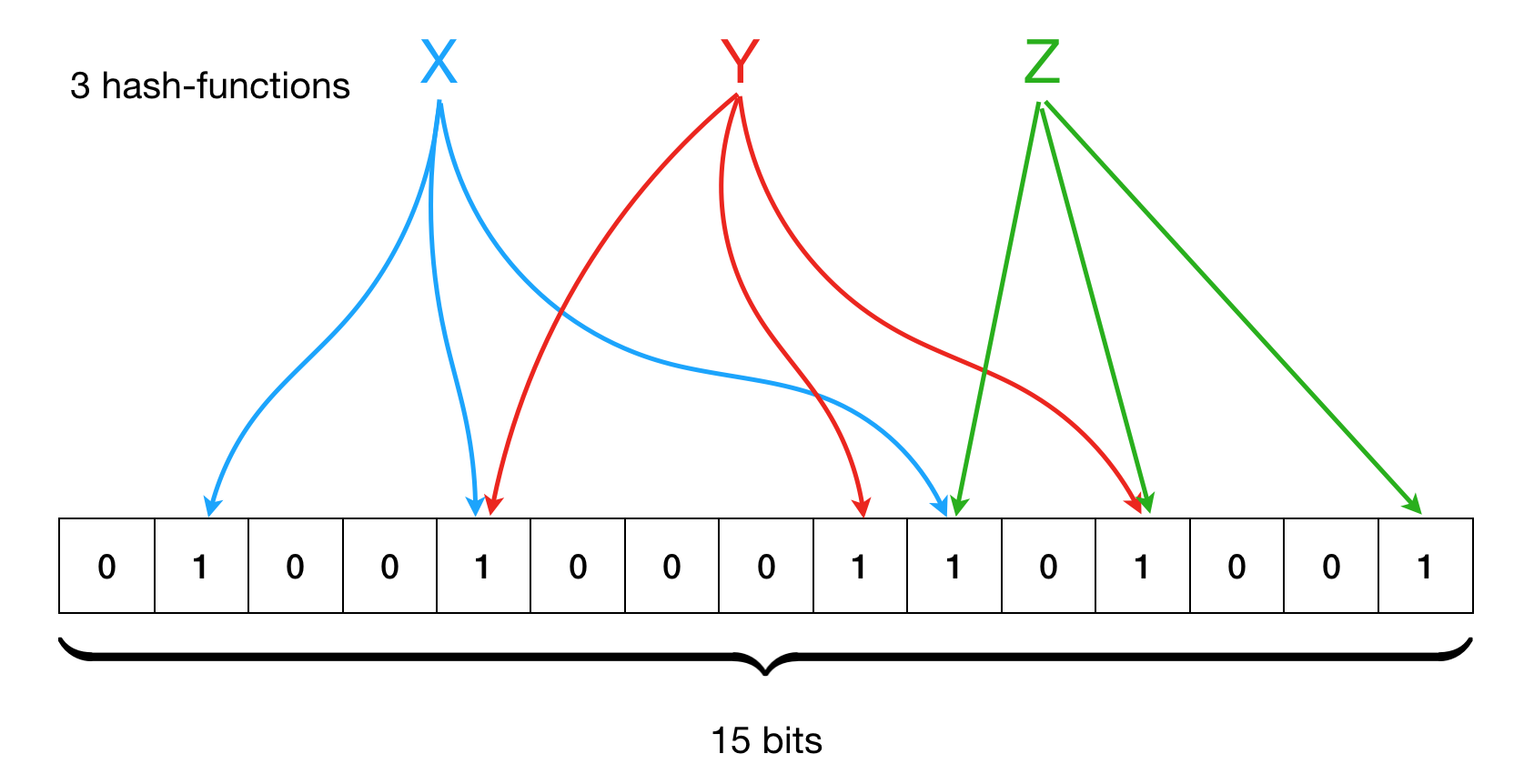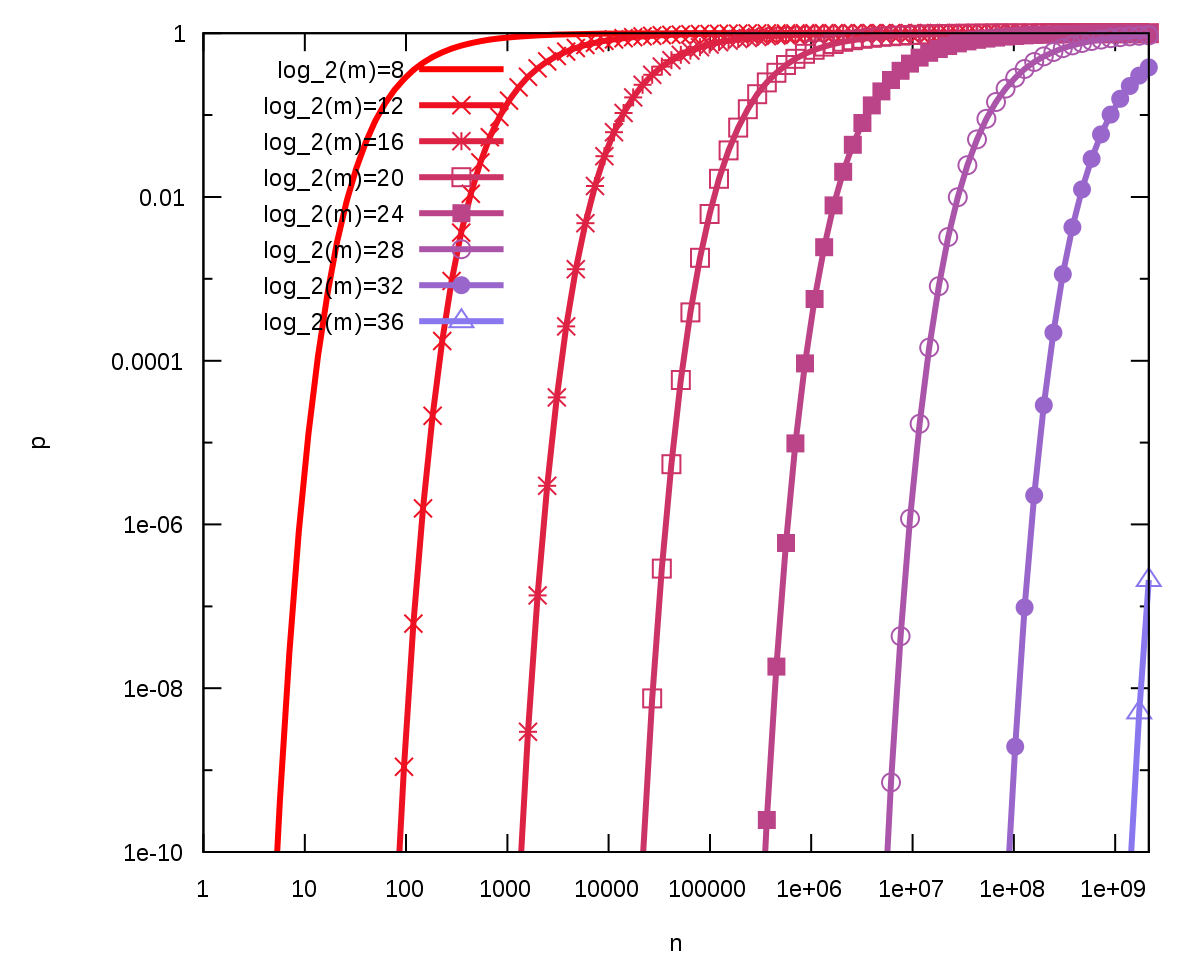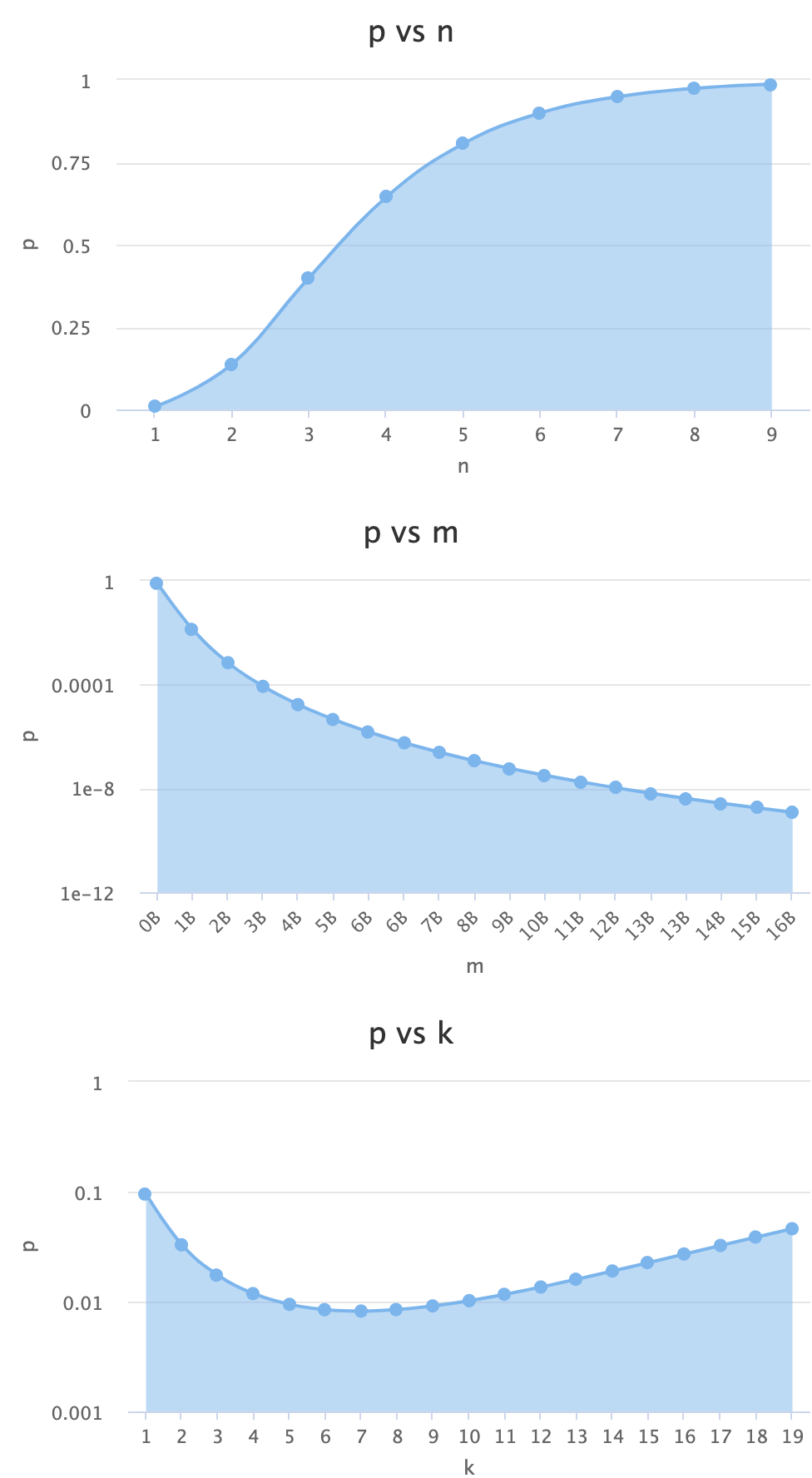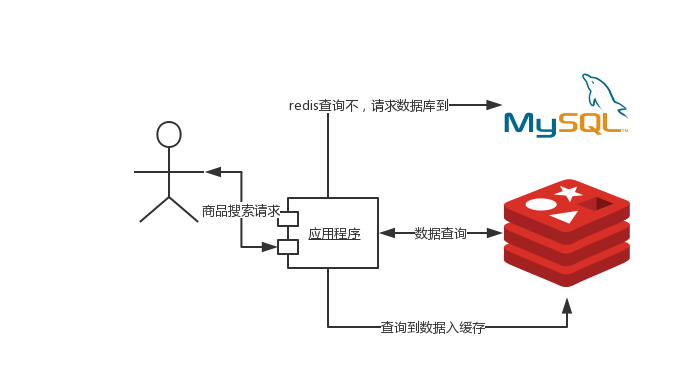## 布隆过滤器

• 使用有限位数组表示大于它长度的元素数量，因为一个位的状态值可以同时标识多个元素。
• 不能删除元素。因为一个位的状态值可能同时标识着多个元素。
• 添加元素永远不会失败。只是随着添加元素增多，误判率会上升。
• 如果判断元素不存在，那么它一定不存在。## 数学关系

### 误判概率• $m$ 是位数组的大小；
• $n$ 是已经添加元素的数量；
• $k$ 是哈希函数数量；
• $e$ 数学常数，约等于2.718281828。• 估算最佳布隆过滤器长度。
• 估算最佳哈希函数数量。

### 最佳布隆过滤器长度### 最佳哈希函数数量## 实现布隆过滤器

• 预期添加元素的最大数量。
• 业务对错误的容忍程度。比如1000个允许错一个，那么误判概率应该在千分之一内。

Java中有一些不错的布隆过滤工具包。

• GuavaBloomFilter
• redissonRedissonBloomFilter 可以redis 中使用。

 static <T> BloomFilter<T> create(
Funnel<? super T> funnel, long expectedInsertions, double fpp, Strategy strategy) {
/**
* expectedInsertions：预期添加数量
* fpp：误判概率
*/
long numBits = optimalNumOfBits(expectedInsertions, fpp);
int numHashFunctions = optimalNumOfHashFunctions(expectedInsertions, numBits);
try {
return new BloomFilter<T>(new BitArray(numBits), numHashFunctions, funnel, strategy);
} catch (IllegalArgumentException e) {
throw new IllegalArgumentException("Could not create BloomFilter of " + numBits + " bits", e);
}
}



static long optimalNumOfBits(long n, double p) {
if (p == 0) {
p = Double.MIN_VALUE;
}
return (long) (-n * Math.log(p) / (Math.log(2) * Math.log(2)));
}


static int optimalNumOfHashFunctions(long n, long m) {
return Math.max(1, (int) Math.round((double) m / n * Math.log(2)));
}


redissonRedissonBloomFilter 计算方法也是一致。

    private int optimalNumOfHashFunctions(long n, long m) {
return Math.max(1, (int) Math.round((double) m / n * Math.log(2)));
}

private long optimalNumOfBits(long n, double p) {
if (p == 0) {
p = Double.MIN_VALUE;
}
return (long) (-n * Math.log(p) / (Math.log(2) * Math.log(2)));
}


## 内存占用

expected HashSet fpp=0.0001 fpp=0.0000001
100万 18.28MB 2.29MB 4MB
1000万 182.82MB 22.85MB 40MB
1亿 1.78G 228.53MB 400MB## 应用场景

1. 弱密码检测；
2. 垃圾邮件地址过滤。
3. 浏览器检测钓鱼网站；
4. 缓存穿透。

### 缓存穿透

1. 数据库不命中，空结果不会写回缓存并返回空结果。
2. 数据库命中，查询结果写回缓存并返回结果。## 小结

https://en.wikipedia.org/wiki/Bloom_filter

https://hur.st/bloomfilter